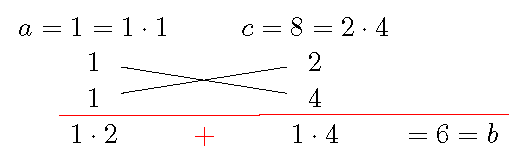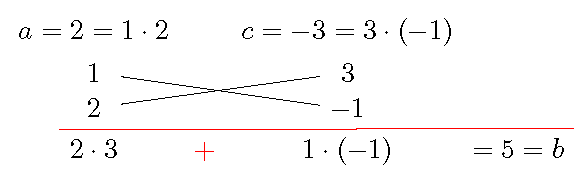# Topic 2 Review of Factoring

## 2.1 Can You Beat a Calculator

Do you know a faster way to find the values?

1. Find the value of the polynomial $$2x^3-98x$$ when $$x=-7$$.
2. Find the value of the polynomial $$x^2-9x-22$$ when $$x=11$$.
3. Find the value of the polynomial $$x^3-2x^2-9x+18$$ when $$x=-3$$.
4. Find the value of $$16^2-14^2$$.

## 2.2 Factor by Removing the GCF

The greatest common factor (GCF) of two terms is a polynomial with the greatest coefficient and of the highest possible degree that divides each term.

To factor a polynomial is to express the polynomial as a product of polynomials of lower degrees. The first and the easiest step is to factor out the GCF of all terms.

Example 2.1 Factor $$4x^3y-8x^2y^2+12x^3y^3$$.

Solution.

1. Find the GCF of all terms.
The GCF of $$4x^3y$$, $$-8x^2y^2$$ and $$12x^4y^3$$ is $$4x^2y$$.
2. Write each term as the product of the GCF and the remaining factor.
$$4x^3y=(4x^2y)\cdot x$$, $$-8x^2y^2=(4x^2y)\cdot (-2y)$$, and $$12x^4y^3=(4x^2y)(3xy^2)$$.
3. Factor out the GCF from each term.
$$4x^3y-8x^2y^2+12x^3y^3=4x^2y\cdot(x-2y+3xy^2)$$.

## 2.3 Factor by Grouping

For a four-term polynomial, in general, we will group them into two groups and factor out the GCF for each group and then factor further.

Example 2.2 Factor $$2x^2-6xy+xz-3yz$$.

Solution.

For a polynomial with four terms, one can normally try the grouping method.

1. Group the first two terms and the last two terms. \begin{aligned} &2x^2-6xy+xz-3yz\\ =&(2x^2-6xy)+(xz-3yz) \end{aligned}
2. Factor out the GCF from each group.
\begin{aligned} =&2x(x-3y)+z(x-3y) \end{aligned}
3. Factor out the binomial GCF. \begin{aligned} =&(x-3y)(2x+z). \end{aligned}

Example 2.3 Factor $$ax+4b-2a-2bx$$.

Solution.

1. Group the first term with the third term and group the second term with the last term. \begin{aligned} &ax+4b-2a-2bx\\ =&(ax-2a)+(-2bx+4b) \end{aligned}
2. Factor out the GCF from each group. \begin{aligned} =&a(x-2)+(-2b)(x-2) \end{aligned}
3. Factor out the binomial GCF. \begin{aligned} =&(x-2)(a-2b). \end{aligned}

Guess and check.

Once you factored one group, you may expect that the other group has the same binomial factor so that factoring may be continued.

## 2.4 Factor Difference of Powers

Factoring is closely related to solving polynomial equations. If a polynomial equation $$p(x)=0$$ has a solution $$r$$, then $$p(x)$$ has a factor $$x-r$$. For example, $$x^n-r^n=0$$ has a solution $$x=r$$. So the difference $$x^n-r^n$$ has a factor $$(x-r)$$. Using long division or by induction, we obtain the following equality.

Difference of $$n$$-th powers

$a^n-b^n=(a-b)(a^{n-1}+a^{n-2}b+\cdots +ab^{n-2}+b^{n-1})$

In particular,

$a^2-b^2=(a-b)(a+b).$

Example 2.4 Factor $$25x^2-16$$.

Solution.

1. Recognize the binomial as a difference of squares. \begin{aligned} &25x^2-16\\ =&(5x)^2-4^2 \end{aligned}
2. Apply the formula. \begin{aligned} =&(5x-4)(5x+4). \end{aligned}

Example 2.5 Factor $$32x^3y-2xy^5$$ completely.

Solution.

\begin{aligned} 32x^3y-2xy^3 =&2xy(16x^2-y^4)\\ =&2xy((4x)^2-(y^2)^2)\\ =&2xy(4x+y^2)(4x-y^2). \end{aligned}

## 2.5 Factor Trinomials

If a trinomial $$ax^2+bx+c$$, $$A\neq 0$$, can be factored, then it can be expressed as a product of two binomials:
$ax^2+bx+c=(mx+n)(px+q).$ By simplify the product using the FOIL method and comparing coefficients, we observe that
$a=\underbrace{mn}_{\mathrm{F}}\quad\quad\quad b=\underbrace{mq}_{\mathrm{O}}~\underset{+}{\underset{}{+}}~\underbrace{np}_{\mathrm{I}} \quad\quad\quad c=\underbrace{nq}_{\mathrm{F}}$

A trinomial $$ax^2+bx+c$$ is also called a quadratic polynomial. The function defined by $$f(x)=ax^2+bx+c$$ is called a quadratic function.

Trial and error.

The observation suggests to use trial and error to find the undetermined coefficients $$m$$, $$n$$, $$p$$, and $$q$$ from factors of $$a$$ and $$c$$ such that the sum of cross products $$mq+np$$ is $$b$$. A diagram as shown in the following examples will be helpful to check a trial.

Example 2.6 Factor $$x^2+6x+8$$.

Solution. One may factor the trinomial in the following way.

1. Factor $$a=1$$: $1=1\cdot 1.$

2. Factor $$c=8$$: $8=1\cdot 8=2\cdot 4.$

3. Choose a proper combination of pairs of factors and check if the sum of cross product equals $$b=6$$: $1\cdot 4+ 1\cdot 2=6.$
This step can be checked easily using the following diagram.4. Factor the trinomial $x^2+6x+8=(x+2)(x+4).$

Example 2.7 Factor $$2x^2+5x-3$$.

Solution. One may factor the trinomial in the following way.

1. Factor $$a=2$$: $1=1\cdot 2.$

2. Factor $$c=-3$$: $-3=1\cdot (-3)=(-1)\cdot 3.$

3. Choose a proper combination of pairs of factors and if the sum of cross products equals $$b=5$$:
$2\cdot 3+1\cdot(-1)=5.$
This step can be checked easily using the following diagram.4. Factor the trinomial $2x^2+5x-3=(x+3)(2x-1).$

Use Auxiliary Problem.

Some higher degree polynomials may be rewrite as a trinomial after a substitution. Factoring the trinomial helps factor the polynomial.

Example 2.8 Factor the trinomial completely.

$4x^4-x^2-3$

Solution. One idea is to use a substitute.

1. Let $$x^2=y$$. Then $$4x^4-x^2-3=4y^2-y-3$$.
2. Factor the trinomial in $$y$$: $$4y^2-y-3=(4y+3)(y-1)$$.
3. Replace $$y$$ by $$x^2$$ and factor further. $\begin{split} 4x^4-x^2-3&=4y^2-y-3\\ &=(4y+3)(y-1)\\ &=(4x^2+3)(x^2-1)\\ &=(4x^2+3)(x-1)(x+1). \end{split}$

## 2.6 Practice

Problem 2.1 Factor out the GCF.

1. $$18x^2y^2-12xy^3-6x^3y^4$$
2. $$5x(x-7)+3y(x-7)$$
3. $$-2a^2(x+y)+3a(x+y)$$

Problem 2.2 Factor by grouping.

1. $$12xy-10y+18x-15$$
2. $$12ac-18bc-10ad+15bd$$
3. $$5ax-4bx-5ay+4by$$

Problem 2.3 Factor completely.

1. $$25x^2-4$$
2. $$8x^3-2x$$
3. $$25xy^2+x$$

Problem 2.4 Factor completely.

1. $$3x^3+6x^2-12x-24$$
2. $$x^4+3x^3-4x^2-12x$$

Problem 2.5 Factor the trinomial.

1. $$x^2+4x+3$$
2. $$x^2+6x-7$$
3. $$x^2-3x-10$$

Problem 2.6 Factor the trinomial.

1. $$5x^2+7x+2$$
2. $$2x^2+5x-12$$
3. $$3x^2-10x-8$$

Problem 2.7 Factor completely into polynomials with integer coefficients.

1. $$x^3-5x^2+6x$$
2. $$4x^4-12x^2+5$$
3. $$2x^3y-9x^2y^2-5xy^3$$

Problem 2.8 Each of trinomial below has a factor in the table. Match the letter on the left of a factor with a the number on the left a trinomial to decipher the following quotation.

$$\dfrac{\phantom{A}}{13}$$$$\dfrac{\phantom{A}}{10~~2~~9~~15}$$,$$\dfrac{\phantom{A}}{9~~5~~14}$$$$\dfrac{\phantom{A}}{13}$$$$\dfrac{\phantom{A}}{4~~3~~15~~7~~2~~1}$$; $$\dfrac{\phantom{A}}{13}$$$$\dfrac{\phantom{A}}{11~~2~~2}$$,$$\dfrac{\phantom{A}}{9~~5~~14}$$$$\dfrac{\phantom{A}}{13}$$$$\dfrac{\phantom{A}}{8~~5~~3~~6}$$; $$\dfrac{\phantom{A}}{13}$$$$\dfrac{\phantom{A}}{14~~3}$$,$$\dfrac{\phantom{A}}{9~~5~~14}$$$$\dfrac{\phantom{A}}{13}$$$$\dfrac{\phantom{A}}{12~~5~~14~~2~~15~~11~~1~~9~~5~~14}$$.”

 A: $$3x-2$$ B: $$2x+1$$ C: $$x+6$$ D: $$x+7$$ E: $$2x-1$$ F: $$3x-1$$ G: $$x+10$$ H: $$x-8$$ I: $$2x+9$$ J: $$x-1$$ K: $$x+3$$ L: $$2x-5$$ M: $$x+5$$ N: $$x-7$$ O: $$x-13$$ P: $$5x-3$$ Q: $$4x-11$$ R: $$x-9$$ S: $$2x+3$$ T: $$x+4$$ U: $$7x+1$$ V: $$3x+5$$ W: $$3x+4$$ X: $$8x+3$$ Y: $$x-14$$ Z: $$5x-6$$
1. $$x^2-2x-24$$
2. $$6x^2+x-2$$
3. $$x^2-16x+39$$
4. $$6x^2+13x-5$$
5. $$x^2-5x-14$$
6. $$3x^2-5x-12$$
7. $$x^2-x-110$$
8. $$x^2-9$$
9. $$-3x^2+11x-6$$
10. $$x^2-10x+16$$
11. $$-2x^2+5x+12$$
12. $$42x^2-x-1$$
13. $$-2x^2-3x+27$$
14. $$x^2+14x+49$$
15. $$x^2-81$$